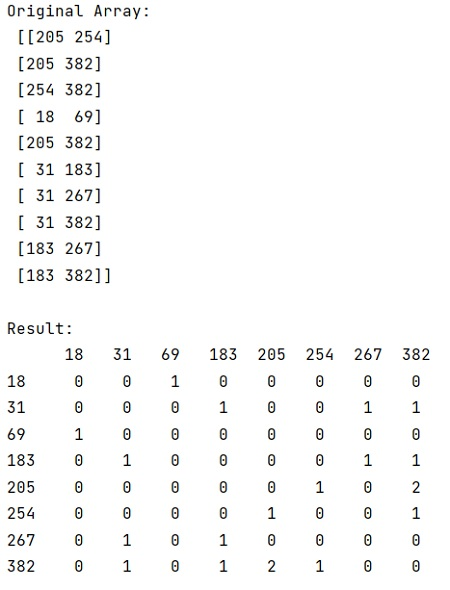# Convert two columns array to matrix with counts of occurrences

NumPy | Two columns array to matrix: In this tutorial, we will learn how to convert an array of two columns to a matrix with counts of occurrences with the help of example in Python NumPy? By Pranit Sharma Last updated : April 25, 2023

Suppose that we are given a numpy array that contains two columns and we need to find a way to transform this array to a symmetric pandas DataFrame that contains the count of occurrences for all possible combinations.

## Convert an array of two columns to a matrix with counts of occurrences

To convert two columns array to a matrix with counts of occurrences, you need to use the networkx package which is used for the creation, manipulation, and study of the structure, dynamics, and functions of complex networks. The use of networkx is to obtain the adjacency matrix directly as a dataframe with nx.to_pandas_adjacency(). To account for the co-occurrences of the edges in the graph, you can create a nx.MultiGraph, which allows for multiple edges connecting the same pair of nodes.

et us understand with the help of an example,

## Python program to convert two columns array to matrix with counts of occurrences

```# Import numpy
import numpy as np

# Import networkx
import networkx as nx

# Creating an array
arr = np.array([(205, 254), (205, 382), (254, 382), (18, 69), (205, 382),(31, 183), (31, 267), (31, 382), (183, 267), (183, 382)])

# Display array
print("Original Array:\n",arr,"\n")

# Transforming array using networkx
res = nx.from_edgelist(arr, create_using=nx.MultiGraph)

# Display Result
print("Result:\n",res,"\n")
```

### Output